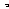# MINLPLib

### A Library of Mixed-Integer and Continuous Nonlinear Programming Instances

#### Instance st_e28

 Formatsⓘ ams gms lp mod nl osil pip py Primal Bounds (infeas ≤ 1e-08)ⓘ -30665.53867000 p1 ( gdx sol ) (infeas: 2e-15) Other points (infeas > 1e-08)ⓘ Dual Boundsⓘ -30665.54004000 (ANTIGONE)-30665.53871000 (BARON)-30665.53907000 (COUENNE)-30665.53867000 (GUROBI)-30665.53868000 (LINDO)-30665.53868000 (SCIP) Referencesⓘ Colville, A R, A Comparative Study of Nonlinear Programming Codes. In Kuhn, H W, Ed, Princeton Symposium on Mathematical Programming, Princeton Univ. Press, 1970.Tawarmalani, M and Sahinidis, N V, Convexification and Global Optimization in Continuous and Mixed-Integer Nonlinear Programming: Theory, Algorithms, Software, and Applications, Kluwer, 2002. Sourceⓘ BARON book instance misc/e28 Added to libraryⓘ 03 Sep 2002 Problem typeⓘ QCQP #Variablesⓘ 9 #Binary Variablesⓘ 0 #Integer Variablesⓘ 0 #Nonlinear Variablesⓘ 5 #Nonlinear Binary Variablesⓘ 0 #Nonlinear Integer Variablesⓘ 0 Objective Senseⓘ min Objective typeⓘ quadratic Objective curvatureⓘ indefinite #Nonzeros in Objectiveⓘ 4 #Nonlinear Nonzeros in Objectiveⓘ 3 #Constraintsⓘ 4 #Linear Constraintsⓘ 1 #Quadratic Constraintsⓘ 3 #Polynomial Constraintsⓘ 0 #Signomial Constraintsⓘ 0 #General Nonlinear Constraintsⓘ 0 Operands in Gen. Nonlin. Functionsⓘ Constraints curvatureⓘ indefinite #Nonzeros in Jacobianⓘ 22 #Nonlinear Nonzeros in Jacobianⓘ 13 #Nonzeros in (Upper-Left) Hessian of Lagrangianⓘ 15 #Nonzeros in Diagonal of Hessian of Lagrangianⓘ 1 #Blocks in Hessian of Lagrangianⓘ 1 Minimal blocksize in Hessian of Lagrangianⓘ 5 Maximal blocksize in Hessian of Lagrangianⓘ 5 Average blocksize in Hessian of Lagrangianⓘ 5.0 #Semicontinuitiesⓘ 0 #Nonlinear Semicontinuitiesⓘ 0 #SOS type 1ⓘ 0 #SOS type 2ⓘ 0 Minimal coefficientⓘ 6.2620e-04 Maximal coefficientⓘ 5.0000e+03 Infeasibility of initial pointⓘ 91.79 Sparsity JacobianⓘSparsity Hessian of Lagrangianⓘ```\$offlisting
*
*  Equation counts
*      Total        E        G        L        N        X        C        B
*          5        4        1        0        0        0        0        0
*
*  Variable counts
*                   x        b        i      s1s      s2s       sc       si
*      Total     cont   binary  integer     sos1     sos2    scont     sint
*         10       10        0        0        0        0        0        0
*  FX      0
*
*  Nonzero counts
*      Total    const       NL      DLL
*         27       11       16        0
*
*  Solve m using NLP minimizing objvar;

Variables  x1,x2,x3,x4,x5,x6,x7,x8,x9,objvar;

Positive Variables  x1,x4;

Equations  e1,e2,e3,e4,e5;

e1..    5*x4 - x5 + 7*x7 - x9 =G= 0;

e2.. -(0.0056858*x6*x9 + 0.0006262*x5*x8 - 0.0022053*x7*x9) + x1 + 2*x4
=E= 85.334407;

e3.. -(0.0071317*x6*x9 + 0.0029955*x5*x6 + 0.0021813*sqr(x7)) + x2 =E= 80.51249
;

e4.. -(0.0047026*x7*x9 + 0.0012547*x5*x7 + 0.0019085*x7*x8) + x3 + 4*x4
=E= 9.300961;

e5.. -(5.3578547*sqr(x7) + 0.8356891*x5*x9 + 37.293239*x5) - 5000*x4 + objvar
=E= -40792.141;

* set non-default bounds
x1.up = 92;
x2.lo = 90; x2.up = 110;
x3.lo = 20; x3.up = 25;
x5.lo = 78; x5.up = 102;
x6.lo = 33; x6.up = 45;
x7.lo = 27; x7.up = 45;
x8.lo = 27; x8.up = 45;
x9.lo = 27; x9.up = 45;

* set non-default levels
x5.l = 78.62;
x6.l = 33.44;
x7.l = 31.07;
x8.l = 44.18;
x9.l = 35.22;

Model m / all /;

m.limrow=0; m.limcol=0;
m.tolproj=0.0;

\$if NOT '%gams.u1%' == '' \$include '%gams.u1%'

\$if not set NLP \$set NLP NLP
Solve m using %NLP% minimizing objvar;

```

Last updated: 2023-08-16 Git hash: 2519540e